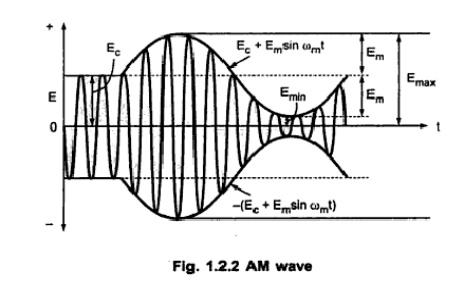Home | | Analog and Digital Communication | Theory of Amplitude Modulation

# Theory of Amplitude Modulation

Amplitude Modulation is the changing the amplitude of the carrier signal with respect to the instantaneous change in message signal.

THEORY OF AMPLITUDE MODULATION

Amplitude Modulation

Amplitude Modulation is the changing the amplitude of the carrier signal with respect to the instantaneous change in message signal.

The amplitude modulated wave form, its envelope and its frequency spectrum and bandwidth.

Fig (a) Sinosoidal modulation signal (b)High frequency carrier (c) AM signal.Using the above mathematical expressions for modulating and carrier signals, we can create a new mathematical expression for the complete modulated wave. It is given as,Modulation Index and Percent Modulation

The ratio of maximum amplitude of modulating signal to maximum amplitude carrier signal is called modulation index. i.e.,Value of Em must be less than value of Ec to avoid any distortion in the modulated signal. Hence maximum value of modulation index will be equal to 1 when Em=Ec. Minimum value will be zero. If modulation index is higher than 1, then it is called over modulation. Data is lost in such case. When modulation index is expressed in percentage , it is also called percentage modulation.

Calculation of modulation index from AM waveform:

Fig 1.2.2 shows the AM waveform. This is also called time domain representation of AM signal.It is clear from the above signal that the modulating signal rides upon the carrier signal. From above figure we can write,Frequency Spectrum and Bandwidth

The modulated carrier has new signals at different frequencies, called side frequencies or sidebands. They occur above and below the carrier frequency.Consider the expression of AM wave given by equation (1.2.3),I.e.,From this equation we can prepare the frequency spectrum of AM wave as shown below in fig. 1.2.3.Fig:1.2.3 : Frequency domain Representation of AM Wave

This contains full carrier and both the sidebands, hence it is also called Double Sideband Full Carrier (DSBFC) system. We will be discussing this system, its modulation circuits and transmitters next, in this section.

We know that bandwidth of the signal can be obtained by taking the difference between highest and lowest frequencies. From above figure we can obtain bandwidth of AM wave as,Amplitude Modulation of Power distribution:

AM Power Distribution:

AM signal has three components : Unmodulated carrier, lower sideband and upper sideband. Hence total power of AM wave is the sum off carrier power Pe and powers in the two sidebands PLSB. i.e.,Here all the three voltages are rms values and R is characteristic impedence of antenna in which the power is dissipated. The Carrier Poweer is,This equation relates total power of AM wave to carrier power, Maximum Value of modulation index, m=1 to avoid distortion. At this value of modulation index, Ptotal = 1.5 Pc. From the above equation we haveExample Problems:Study Material, Lecturing Notes, Assignment, Reference, Wiki description explanation, brief detail
Analog and Digital Communication : Theory of Amplitude Modulation |